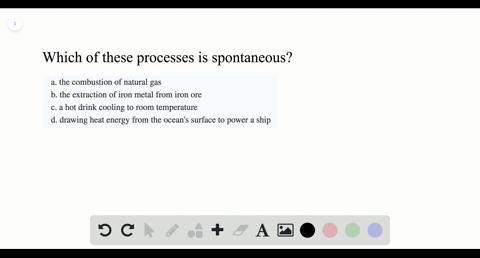### Which of these processes is spontaneous? \begin{…

00:56
LP
Problem 26

How does the value of $\Delta G^{\circ}$ for a reaction reaction relate to the equilibrium constant for the reaction? What does a negative $\Delta G^{\circ}$ for a reaction imply about $K$ for the reaction? A positive $\Delta G^{\circ} ?$

The relationship between $\Delta G^{\circ}$ and $K$ is logarithmic. Small changes in $\Delta G^{\circ}$
have a large effect on $K .$ If the reactants undergo a large negative Gibbs
free energy change as they become products, then the reaction will have a large equilibrium constant, with products strongly favored at equilibrium.
If the reactants undergo a large positive Gibbs free energy change as they
become products, then the reaction will have a small equilibrium constant,
with reactants strongly favored at equilibrium.

## Discussion

You must be signed in to discuss.

## Video Transcript

So for reaction. There's an equivalent equilibrium Constant called Kay, and we won't know how that relates to Delta G for the reaction. So don't the G equals minus R T fell in, Okay? And so that's the relationship that used to have in this equation can be found in your textbook. I won't get through the purpose of deriving this. And so what? The question also wants to know is it's Delta G understanding conditions. They want to know how the sign of Delta G effects that what K is. So let's think about the sign of these different numbers are is a constant, and it's positive. Tia's temperature and it's a variable. But it could never be negative if you're considering it in terms of Kelvin, so we'll consider it positive as well. And so now when you think about what? Don't geek me positive. Negative. So it's considered a case at Delta G is positive. If Delta G is positive, you have a negative times of positive times positive, so you need similar negatives, so this only cape out. You must be negative. And how does that happen? That happens when Kay is less than one. So don't The G It's positive. Okay? Is less than one. The other case, don't that she is negative. So you still have a negative times? Positive times, a positive. And so you need okay to be positive. And so this happens when Kay is greater than one. So in Delta G is negative, Okay, Is greater than one. And so that shows you how the sign of Dr G relates to what Caisse?